### Which AWS EC2 Instance Type Will Work Best for your Load Test?

Before performing a load test, you need to determine the right AWS EC2 instance type. Instance types comprise various combinations of CPU, memory, storage, and networking capacity and give you the flexibility to choose the appropriate mix of resources for your applications. There of course cost implications for which we discus estimation of those in a related  post.  In this blog post we will see how load testing with different AWS EC2 instance types can impact your load testing results, sometimes in surprising ways.

More importantly, you can repeat this with your own test scripts and different instance types and find what is best for you!

### Introduction

We will perform load tests on different AWS EC2 instance sizes and compare the results. We will keep other configuration settings constant, such as AWS locations, so that we don’t introduce other variables. The results will give us something to think about when selecting the right instances for future load testing.

Our example load test will be to sort a large array. To do this we will generate 100,000 numbers and save it on a file and then implement a “Heap Sort” algorithm to sort the numbers. Lastly we will run the script on different instances sizes in one load test at the same time. We are setting the thread count to 100 and will run the test with RedLine13. We would expect better hardware to produce better results. However at the end of the testing, a little surprise may come up.

### Let’s start

First, we used a free site to generate 100,000 random numbers and saved it. We did this by selecting “Make a list of numbers starting at” 1 and ending at 100,000 and clicked “Generate List of Numbers”. After generating the numbers we downloaded the txt file.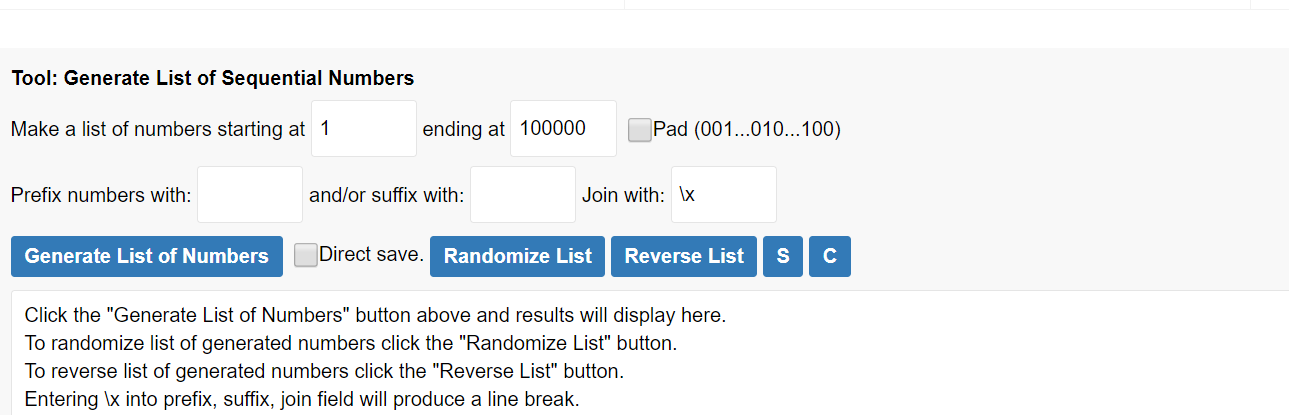We needed to extract the AWS instance type size and pass this as a variable to name our action in the Sorting algorithm. To do this, we can use 2 different methods. One of them is OSSampler and the other is the JSR223 Sampler on Apache JMeter. In this example, we used the JSR223 Sampler to execute a command on AWS instances. As a sidenote, we will create a separate blog post about How to get AWS Meta Information soon.

Let’s add the JSR223 Sampler to our Thread Group and add the script to execute a Linux command on an EC2 instance to get the instance type.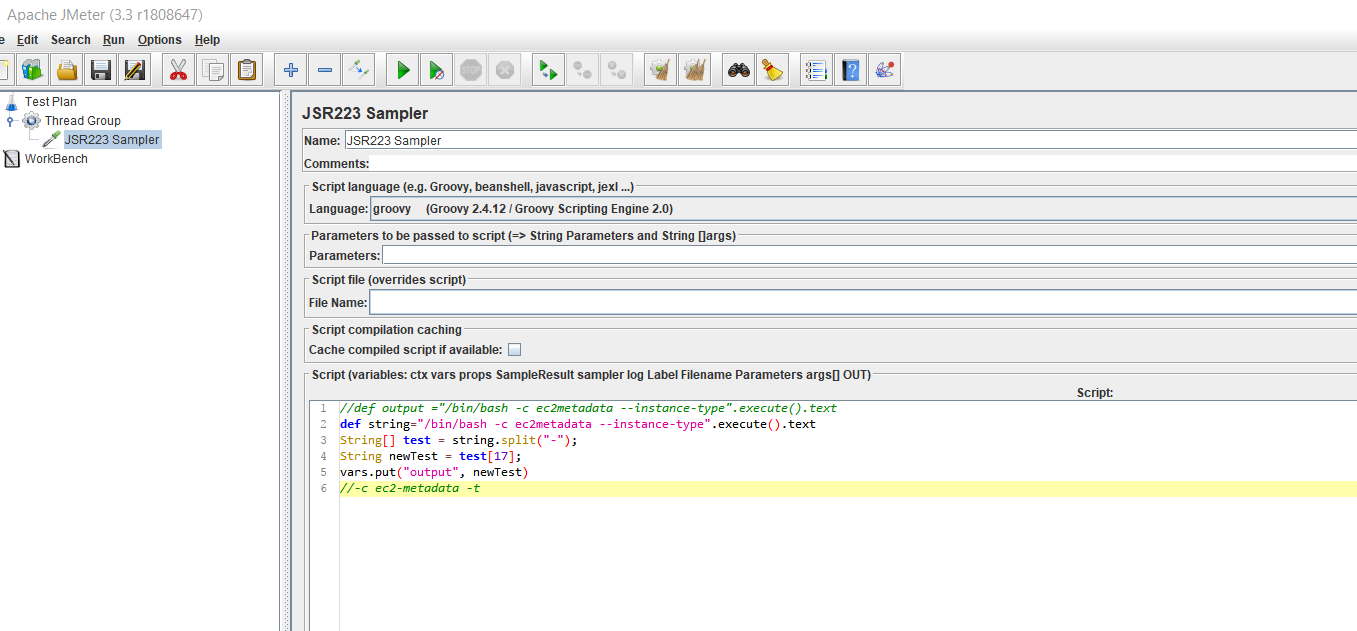After executing the script, the important point is that we need to save the AWS instance name as a variable. Our variable name is “output”.

Now, we add a BeanShell Sampler on our Thread Group to execute Merge sorting operations. The crucial part of this section is giving the sampler a distinguishing name. Our distinguishing name is the AWS instance name which we extracted from our previous sampler (“output”). So we are naming this sampler as BeanShell `\${output}`. `\${output}` refers to AWS EC2 instance type name such as a m4.largelocal.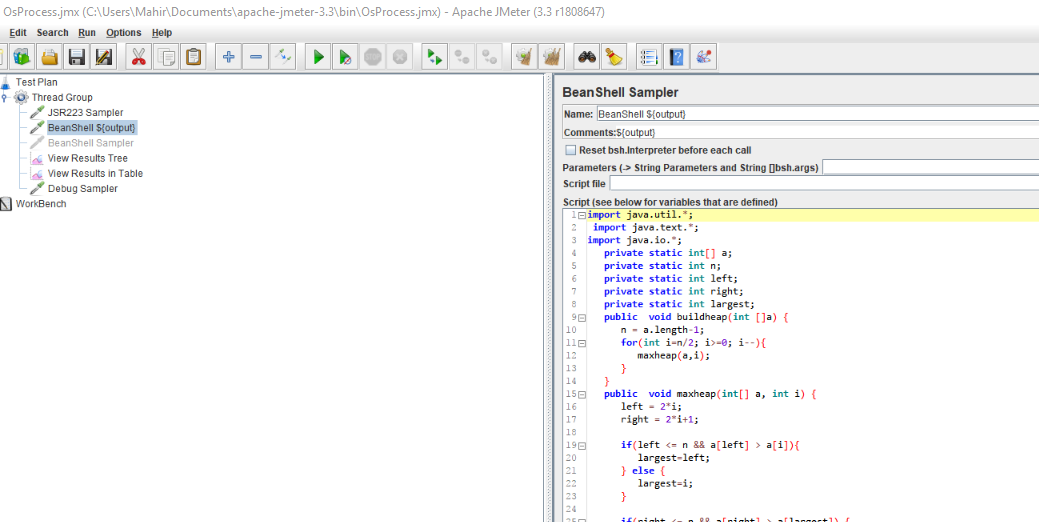After completing the script, we are now running the script on various AWS EC2 instances at the same time. With RedLine13, adding an EC2 server is extremely easy and flexible. Click Servers → Server Mgmt → Add Server and then choose the instances.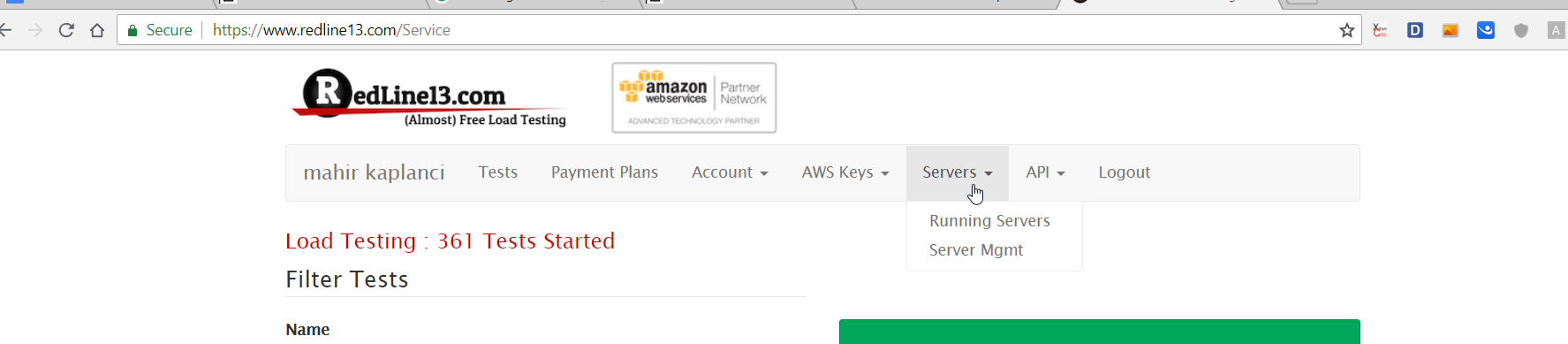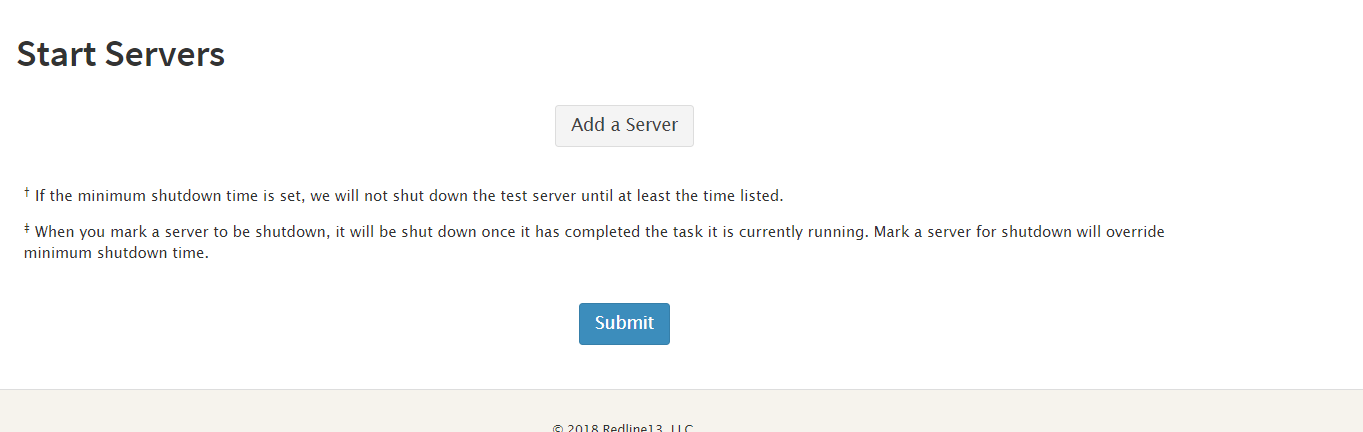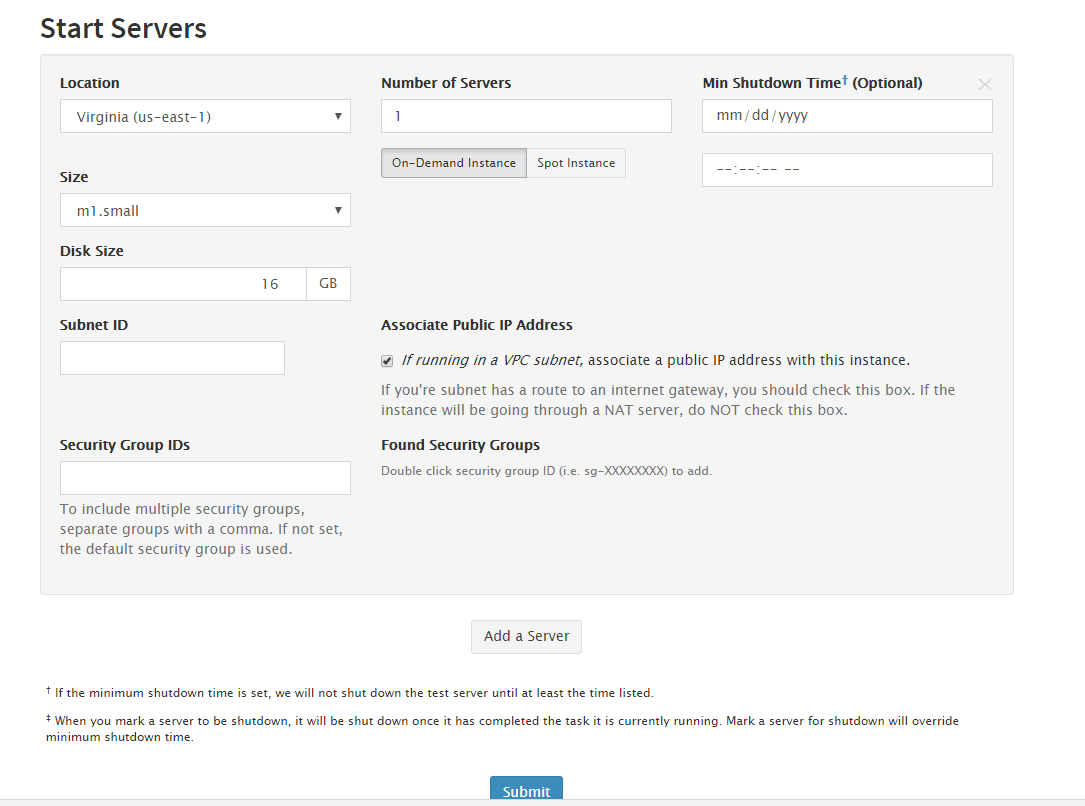### Load Test Results with Different AWS EC2 Instance Types

In our example we have picked 5 different instance types:

1. m3.medium
2. m4.large
3. t2.2xlarge
4. t2.medium
5. t2.nano

After launching these servers, let’s run the our script on RedLine13. The results are shown below and it gave us unexpected results.After this test, our initial thought is that we won’t use m3.medium instance type for load testing. However, as we stated above, different load tests will behave differently on different AWS EC2 instance types. So the takeaway is to look at load testing with different AWS EC2 instance types by trying your load test on different instance types and determine which is best for you.

### Beanshell Sampler Java Code

```def string = "/bin/bash -c EC2metadata --instance-type".execute().text

String[] test = string.split("-");
String newTest = test;
vars.put("output", newTest)

import java.util.*;
import java.text.*;
import java.io.*;

private static int[] a;
private static int n;
private static int left;
private static int right;
private static int largest;

public void buildheap(int[] a) {
n = a.length - 1;
for (int i = n / 2; i >= 0; i--) {
maxheap(a, i);
}
}

public void maxheap(int[] a, int i) {
left = 2 * i;
right = 2 * i + 1;

if (left <= n && a[left] > a[i]) {
largest = left;
} else {
largest = i;
}

if (right <= n && a[right] > a[largest]) {
largest = right;
}

if (largest != i) {
exchange(i, largest);
maxheap(a, largest);
}
}

public void exchange(int i, int j) {
int t = a[i];
a[i] = a[j];
a[j] = t;
}

public void sort(int[] myarray) {
a = myarray;
buildheap(a);
for (int i = n; i > 0; i--) {
exchange(0, i);
n = n - 1;
maxheap(a, 0);
}
}

String csvTest = "numbers.txt";
ArrayList strList = new ArrayList();
File file = new File(System.getProperty("user.dir") + File.separator + csvTest);
if (!file.exists()) {
throw new Exception("ERROR: file " + csvTest + " not found in " + csvDir + " directory.");
}

int[] arr = new int;
String line = null;
int i = 0;
while ((line = bufRdr.readLine()) != null) {